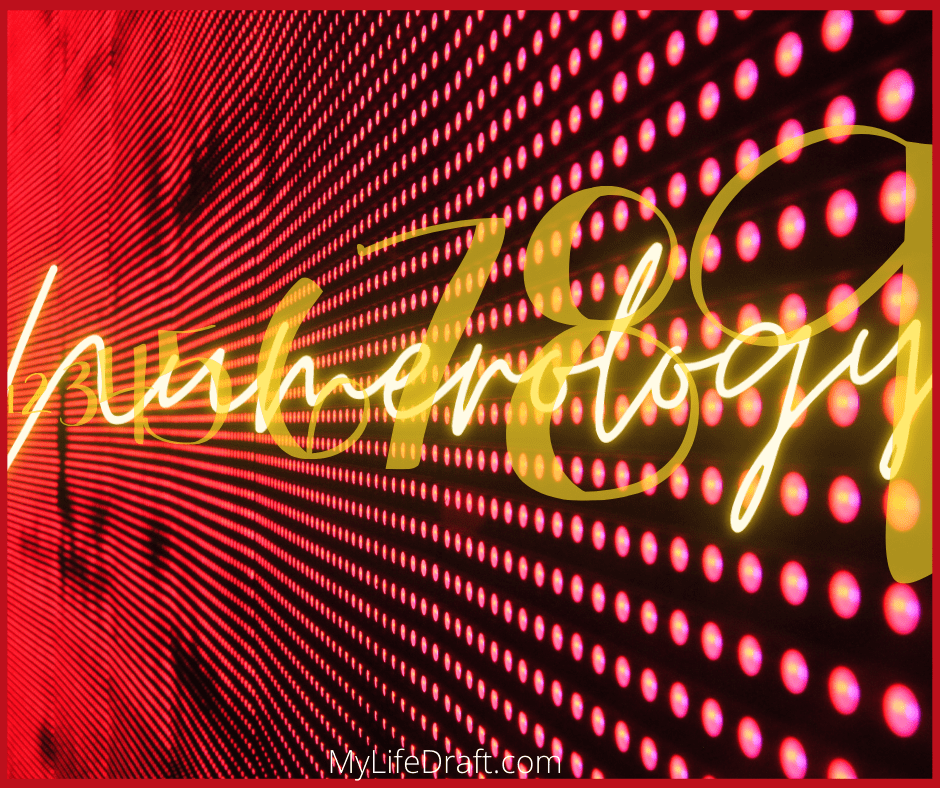# What Numerology Numbers Mean

Spread the love

Explore the ancient craft of what Numerology Numbers mean and reveal your life path and destiny. Numbers give you insight into the life you are living now and a pathway to follow to happiness.

“Overall, delving into the world of numbers will provide you with a simple and accurate way to decipher your experiences in the same manner that a road map helps you navigate a route that you haven’t previously travelled.” ~David A. Phillips

First, Numerology is a cool science that connects you to numbers from the time you were born and the name you were given at birth. To be connected in such a way is truly incredible. This guidance improves your life, so you feel better about tackling big problems, and the little ones too.

We could all use some help when it comes to what our future looks like. If you are having a hard time and want some assistance in your life, keep reading. Therefore let’s start with the basics here and analyze your birthdate.

## What is your life path number in Numerology?

Your life path number is a number that helps you witness what your growth is and where to go from there. it reveals what your strengths are and what your numerology number means. Your life path number also summarises where you are now. It may not feel good to look back if you have a bad past, however, numerology analyzes that too.

If you would like to know more about your life path number, I’ve written about Life Path numerous times, including what each number means.

## This is Numerology, and this is My Numbers?

If you were born on November 6, 1983, this is how you figure out your life path number. In the process, we always narrow down to a single-digit number.

November is the eleventh month (11), Six is the day you were born (6), and 1986 is the year you were born (1+9+8+3). This means that you add the month and 11 (1+1)=2. Then add the day (+6)which equals (2+6)=8. Then add the year’s sum and your get 21. (2+1)=3.

Our equation is now (8+3)= 11 and the single-digit number is (1=1)= 2.

As we can see, 2 is My Life Path Number in numerology is very nurturing. A quick look also shows life path number 2, representing a compassionate and helpful person. They are exceptional at forming relationships. Affectionate and always considers people’s feelings.

If you would like more on other life path numbers go here.

### What is the Destiny Number?

The Destiny Number or Expression Number is the number that shows you what your future is. What your future hold for your and you can analyze this to see if you are on the right path. You may be at a crossroads and this number can help lead you in the right direction so you are happy.

The Destiny Number is figured out by a chart using your name at birth. There are two types of charts the Chaldean and Pythagorean charts. The most common chart is the Pythagorean chart and looks like the photo below.

### The Pythagorean Numerology Chart

Let’s find the Destiny Number or Expression Number for the name Willow Jane White in the Pythagorean Numerology Chart. To do this, we line up each letter in the row with the number and add as we did in the previous with the Life Path number.

Willow is the first name, and it would look like this. (5+9+3+3+6+5)=31 (3+1)=4

Jane, the middle name would look like this (1+1+5+5)= 12(don’t forget to always reduce to a single digit). (1+2)=3

White reduced would look like this following the chart. (5+8+9+2+5)=29 (2+9)=11 (1+1)=2

After all the letters are in the numerical value, you add them all up. So from Full The Name, Willow (4) Jane (3) and White (2) (4+3+2)=9 Now we can see that the Destiny/Expression Number is the number 9.

If you use the Chaldean chart as shown below, it is different looking but the same idea—just a different method in the number and letters.

### The Chaldean Numerology Chart

So let’s do the figuring for The Destiny or Expression Number in the Chaldean Numerology Chart. It is done the same way, and we will use the same name.

So, Willow Jane White is the full name at birth, and Willow looks like this in numerical values. (6+1+3+3+7+6)=26 (2+6)=8

And Jane, the middle name would look like this. (1+1+5+5)=12(Again, don’t forget to reduce to a single digit.) (1+2)=3

The last name White reduced would look like this following the Chaldean chart. (6+5+1+4+5)=21 (2+1)=3

When all the letters are in the numerical value, you add them all up. So from Full The Name, Willow (8) Jane (3) and White (3) (8+3+3)=14 reduced to a single digit (1+4)=5 Now we can see that the Destiny/Expression Number is the number 5.

What numerology numbers mean if you would like to know what each number means, Check out my blog on what does each number means in numerology.This site uses Akismet to reduce spam. Learn how your comment data is processed.